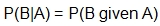# Student Resources

## Glossary

Conditional probability
The probability of an event happening, given that another event has already happened.= the probability of event B happening given that event A happens

Dependent event
An event that is affected by a previous event. The outcome of the event is affected by the outcome of the previous event.

Experimental probability
The ratio of the number of times an event occurs to the total number of trials or times the activity is performed. It is the estimate of an event occurring, based on the results of observations or results of an experiment.

Independent event
An event that is not affected by a previous event. The outcome of the event is not affected by the outcome of the previous event.

Theoretical probability
The number of ways that the event can occur, divided by the total number of outcomes.